# 旅游业与区域经济增长—基于云南省的时间序列数据Tourism and Regional Economic Growth—Time Series Data Based on Yunnan Province

DOI: 10.12677/ASS.2018.75110, PDF, HTML, XML, 下载: 580  浏览: 923

Abstract: This paper reviews the literature on tourism and economic growth at home and abroad, analyzes the deficiencies of existing researches, and analyzes the impact of tourism on the economic growth of Yunnan province. At the same time using the unit root test, E-G two step cointegration model and granger causality test, based on 2000-2017 in Yunnan province tourism revenue, GDP as the proxy variable of time series data of Yunnan province as the research object, the long-term equilibrium economic growth and the development of tourism and causal relationship. The results showed that: 1) Economic growth and tourism development in Yunnan province has a stable cointegration relationship for a long time, and GDP growth to the development of the tourism industry of irreversible one-way granger causality relationship. 2) From the ECM model, it can be learned that the impact of tourism on GDP in Yunnan province is more significant than that in the short term. 3) In a period of time, Yunnan’s tourism industry has been actively promoting the development of local economy, and its efficiency is relatively high. The conclusion provides important policy implications for the coordinated development of Yunnan economy and tourism.

1. 引言

2. 文献综述

3. 云南省旅游业与经济增长关系的实证研究

3.1. 计量方法及模型

3.1.1. 单位根检验

$\Delta {Y}_{t}=m{Y}_{t-1}+\underset{i=1}{\overset{p}{\sum }}{\beta }_{i}\Delta {Y}_{t-1}+{\mu }_{t},\text{\hspace{0.17em}}\text{\hspace{0.17em}}\text{\hspace{0.17em}}\text{\hspace{0.17em}}t=1,2,\cdots ,T$ (3-1)

$\Delta {Y}_{t}=m{Y}_{t-1}+t+\underset{i=1}{\overset{p}{\sum }}{\beta }_{i}\Delta {Y}_{t-1}+{\mu }_{t},\text{\hspace{0.17em}}\text{\hspace{0.17em}}\text{\hspace{0.17em}}\text{\hspace{0.17em}}t=1,2,\cdots ,T$ (3-2)

$\Delta {Y}_{t}=m{Y}_{t-1}+t+{\delta }_{t}+\underset{i=1}{\overset{p}{\sum }}{\beta }_{i}\Delta {Y}_{t-1}+{\mu }_{t},\text{\hspace{0.17em}}\text{\hspace{0.17em}}\text{\hspace{0.17em}}\text{\hspace{0.17em}}t=1,2,\cdots ,T$ (3-3)

3.1.2. 协整检验

E-G两步协整检测法：

${Y}_{t}=\beta {X}_{t}+{\mu }_{t}$ (3-4)

${e}_{t}={Y}_{t}-\beta {X}_{t}$ (3-5)

3.1.3. 格兰杰因果关系检验

${Y}_{t}={\alpha }_{1}{Y}_{t-1}+\underset{i=1}{\overset{m}{\sum }}{\beta }_{i}\Delta {Y}_{t-1}+{\mu }_{t}$ (3-6)

${X}_{t}=\underset{i=1}{\overset{m}{\sum }}{\lambda }_{i}\Delta {Y}_{t-1}+\underset{i=1}{\overset{m}{\sum }}{\delta }_{i}{X}_{t-1}+\epsilon$ (3-7)

3.1.4. 误差修正模型(ECM)

Engel-Granger两步法

1) 进行协整回归：

${Y}_{t}={\alpha }_{0}+{\alpha }_{1}{X}_{t}+{\mu }_{t},\text{\hspace{0.17em}}\text{\hspace{0.17em}}\text{\hspace{0.17em}}\text{\hspace{0.17em}}t=1,2,\cdots ,T$ (3-8)

${e}_{t}={Y}_{t}-{\alpha }_{0}-{\alpha }_{1}{X}_{t},\text{\hspace{0.17em}}\text{\hspace{0.17em}}\text{\hspace{0.17em}}\text{\hspace{0.17em}}t=1,2,\cdots ,T$ (3-9)

2) 若变量之间确存在协整关系，则用最小二乘方法进行检验。

3.2. 实证分析

3.2.1. 数据的选择与处理

3.2.2. 数据相关性分析

3.2.3. 单位根检验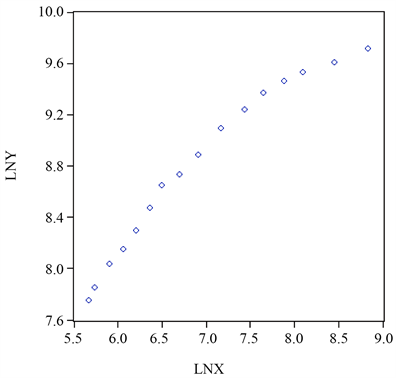Figure 1. Scatter plot based on the time series data of lnX and lnY in Yunnan from 2000 to 2017Table 1. ADF test results of variables lnX, lnY, DlnX, DlnY, DDlnX, DDlnY

3.2.4. E-G两步协整检验Figure 2. Sequence diagram of scatter plots based on the time series data lnX and lnY of Yunnan 2000-2017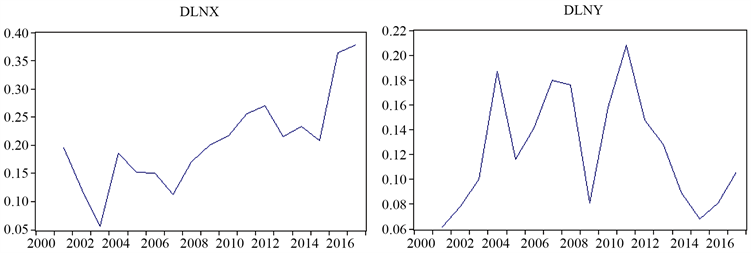Figure 3. Sequence diagram of scatter plot based on the time series data DlnX and DlnY of Yunnan 2000-2017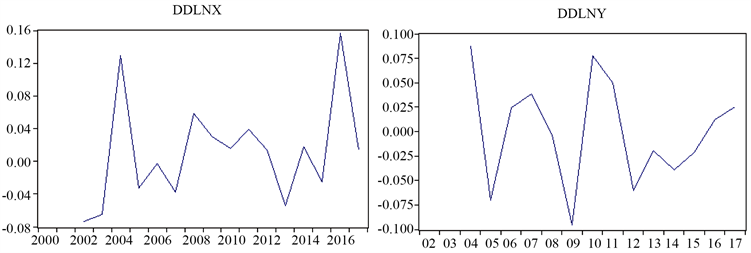Figure 4. Based on the time series of DlnX and DlnY time series data of Yunnan 2000-2017

1) 构建模型。假设上述序列模型为

$\mathrm{ln}{Y}_{t}={\beta }_{0}+{\beta }_{1}\mathrm{ln}{X}_{t}+{\mu }_{t}$ (3-10)

2) 进行回归分析：

$\begin{array}{l}\mathrm{ln}{Y}_{t}=4.131871+0.667123\mathrm{ln}{X}_{t}\\ t=\left(17.430181\right)\text{\hspace{0.17em}}\text{\hspace{0.17em}}\text{\hspace{0.17em}}\text{\hspace{0.17em}}\text{\hspace{0.17em}}\left(16.36798\right)\\ {R}^{2}=0.959092\text{\hspace{0.17em}}\text{\hspace{0.17em}}\text{\hspace{0.17em}}\text{\hspace{0.17em}}\text{D}.\text{W}.=0.253932\end{array}$ (3-11)

${e}_{t}=\mathrm{ln}{Y}_{t}-4.131871-0.667123\mathrm{ln}{X}_{t}$ (3-12)

3.2.5. 格兰杰因果关系检验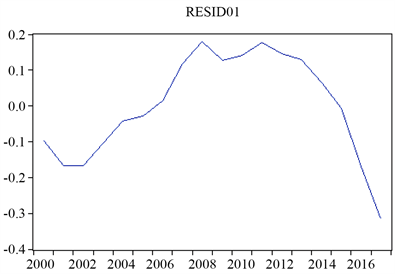Figure 5. Regression equation residual timing diagramTable 2. Mean test results of residual seriesTable 3. Unit root test results for residualsTable 4. Yunnan tourism total income X and Yunnan Province GDP difference and logarithmic processingTable 5. Granger causality test results

3.2.6. 误差修正模型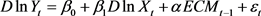(3-13)

$\begin{array}{l}D\mathrm{ln}{Y}_{t}={\beta }_{0}+{\beta }_{1}D\mathrm{ln}{X}_{t}+\alpha EC{M}_{t-1}+{\epsilon }_{t}\\ t=\left(3.452013\right)\text{\hspace{0.17em}}\text{\hspace{0.17em}}\text{\hspace{0.17em}}\text{\hspace{0.17em}}\text{\hspace{0.17em}}\left(9.644895\right)\text{\hspace{0.17em}}\text{\hspace{0.17em}}\text{\hspace{0.17em}}\text{\hspace{0.17em}}\text{\hspace{0.17em}}\left(-6.932606\right)\\ {R}^{2}=0.750872\text{\hspace{0.17em}}\text{\hspace{0.17em}}\text{\hspace{0.17em}}\text{\hspace{0.17em}}\text{\hspace{0.17em}}\text{\hspace{0.17em}}\text{\hspace{0.17em}}\text{D}.\text{W}.=1.108425\end{array}$ (3-14)

3.3. 实证结果分析

1) 变量lnX与lnY之间的协整关系表明旅游业发展与云南省经济增长存在长期稳定的动态均衡关系。由(3-11)可知，前者增长1%，后者将会增长0.66%。由此可见，云南省旅游业发展能较为明显地带动云南省经济的发展。从(3-14)可以看出，造成lnY短期波动的因素由两个方面构成：第一是短期波动，即LnX短期波动所造成的；第二是长期动态均衡，即通过ECM长期调整达到均衡的调整过程。如果短期波动偶发偏离长期均衡状态，则会引起偏差但是这种偏差不会太久。跟随每一个周期(−0.351587)进行调整，并不断修正这个偏差直到它返回到平衡状态为止。

2) 从格兰杰因果检验可以看出，对于旅游业和经济增长，在5%的显著性水平下，旅游业发展是经济增长的格兰杰原因，但经济增长却不是云南旅游业发展的格兰杰原因，云南旅游业和经济发展存在单向因果关系。换句话说，在过去的18年里，旅游业能够较为明显地拉动云南经济增长，而云南经济增长未能明显地拉动旅游业收入的增长。

3) 从ECM模型中可以看出，在短期内，旅游业收入每增加1%，GDP将会提高0.13%，而对于(1)中我们通过计算得到这个数字是0.66%。因此，对于云南省而言，旅游业对其经济的增长长期相较于短期更为显著。与此同时，经济系统具有较强的自我调节功能，并能自发保持动态均衡。如果短期波动偶发偏离长期均衡状态，则会引起偏差但是这种偏差不会太久。跟随每一个周期(−0.351587)进行调整，并不断修正这个偏差直到它返回到平衡状态为止。

4) 经济变量对GDP的推动效率是衡量经济变量对于GDP推动效率的衡量指标。其计算公式为  ：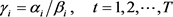(4-15)Table 6. Regression analysis resultsTable 7. Pull efficiency calculation table

4. 政策建议

1) 政府部门在讨论制定当地经济发展和旅游业的计划和政策时，要将经济增长与旅游发展动态平衡和增强前瞻性的发展和长远发展考虑到其中。采取对应措施，以免短期经济波动对于当地旅游行业的冲击，保障当地旅游业和经济增长朝着更好更快的方向发展。

2) 由格兰杰因果检验可知虽然不存在经济增长到云南省旅游业发展的格兰杰原因  ，但这并不能掩盖旅游业对于云南省经济发展的支柱地位，随着云南省旅游业不断地发展旅游发展，云南经济发展也随之不断的变化，其所占云南省GDP的份额也会逐步增大。从而旅游业对于推动云南省经济增长的假设是成立的。随着休闲时代的到来，休闲经济已经成为云南省经济发展的支柱产业。旅游经济的发展是云南省经济发展的战略性结构调整的“指南针”。因此，政府应当将大力发展休闲宜居型，环境友好型，资源节约型的旅游经济发展作为经济发展转型的方式之一。促进云南省接待国内和国际旅游游客的持续、稳定增长以此促进云南经济更好更健康的发展。

3) 旅游支付能力主要是消费者的可支配收入决定，这就要求企业对员工的工资进行提高，但是考虑到云南省的实际情况以及当前我国经济发展的现状，增加工资必须与企业发展与经济发展相适应。但是，在增加工资之外，企业可以考虑对内部员工开设旅游专户的奖励，该项奖励可以作为优秀员工考核绩效奖励，也可以作为年终奖励的部分内容，通过该项奖励不仅可以提高员工的积极性，同时也降低了企业的费用支出。

4) 加强国际旅游吸引力  。云南省地理资源、生物资源丰富，在国际市场上具有不可替代性，但是由于受到出行距离的影响，云南省内的国际旅游大多以东南亚等邻近国家游客为主，且重复旅游的几率较小。因此，云南省应当在此方面加大建设，提高国际旅游吸引力，吸引其他国家，尤其是欧美国家到云南进行旅游消费，增加旅游消费需求的有效性。Table A. Statistics on time series data of Tourism Total Income and GDP in Yunnan Province, 2000-2017

  Gee, C.Y. (1999) International Tourism: A Global Perspective. World Tourism Organization, Madrid.  Gunduz, L. and Hatemi, J.A. (2005) Is the Tourism-Led Growth Hypothesis Valid for Turkey. Applied Economics Letters, 12, 499-504. https://doi.org/10.1080/13504850500109865  Balaguer, L. and Cantavella-Jorda, M. (2002) Tourism as a Long-Run Economic Growth Factor: The Spanish Case. Applied Economics, 34, 877-884. https://doi.org/10.1080/00036840110058923  Oh, C.O. (2005) The Contribution of Tourism Development to Economic Growth in the Korean Economy. Tourism Management, 26, 39-44. https://doi.org/10.1016/j.tourman.2003.09.014  Dritsakis, N. (2004) Tourism as a Long-Run Economic Growth Factor: An Empirical Investigation for Greece Using Causality Analysis. Tourism Economics, 10, 305-316. https://doi.org/10.5367/0000000041895094  Durbarry, R. (2002) The Economic Contribution of Tourism in Mauritius. Annals of Tourism Research, 29, 862-865. https://doi.org/10.1016/S0160-7383(02)00008-7  Kim, H.J., Chen, M.H. and Jang, S.C. (2006) Tourism Expansion and Economic Development: The Case of Taiwan. Tourism Management, 27, 925-933. https://doi.org/10.1016/j.tourman.2005.05.011  李子奈. 计量经济学[M]. 北京: 清华大学出版社, 2000.  罗文斌. 旅游发展与经济增长、第三产业增长动态关系——基于中国1978~2008数据的实证检验[D]: [博士学位论文]. 长沙: 湖南师范大学, 2011.  潘省初. 计量经济学中级教程[M]. 北京: 清华大学出版社, 2013.  云南省统计局. 云南统计年鉴[Z]. 北京: 中国统计出版社, 2017.  陈淑云, 付振奇. 城市化、旅游业与经济增长的关系分析——以湖北省1990~2009年时间序列数据为例[J]. 经济体制改革, 2012(2): 30-35.  廖志豪, 张光远, 吴丽华. 云南旅游产业发展现状及对策研究[J]. 襄樊学院学报, 2004, 25(5): 69-72.  陈友龙, 刘沛林, 许抄军. 我国旅游业发展与经济增长的因果关系研究[J]. 衡阳师范学院学报, 2006, 27(1): 93-97.  宋振丽. 苏南地区旅游产业与经济增长关系实证研究[D]: [硕士学位论文]. 镇江: 江苏大学, 2014.